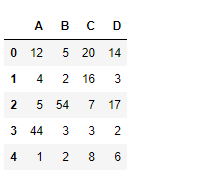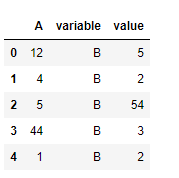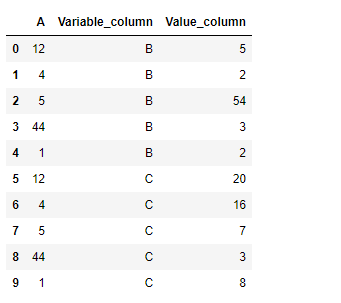Skip to content
Related Articles
Python | Pandas dataframe.melt()
• Last Updated : 24 Nov, 2018

Python is a great language for doing data analysis, primarily because of the fantastic ecosystem of data-centric python packages. Pandas is one of those packages and makes importing and analyzing data much easier.

Pandas` dataframe.melt()` function unpivots a DataFrame from wide format to long format, optionally leaving identifier variables set. This function is useful to massage a DataFrame into a format where one or more columns are identifier variables (id_vars), while all other columns, considered measured variables (value_vars), are “unpivoted” to the row axis, leaving just two non-identifier columns, ‘variable’ and ‘value’.

Syntax:DataFrame.melt(id_vars=None, value_vars=None, var_name=None, value_name=’value’, col_level=None)
Parameters :
frame : DataFrame
id_vars : Column(s) to use as identifier variables
value_vars : Column(s) to unpivot. If not specified, uses all columns that are not set as id_vars.
var_name : Name to use for the ‘variable’ column. If None it uses frame.columns.name or ‘variable’.
value_name : Name to use for the ‘value’ column
col_level : If columns are a MultiIndex then use this level to melt.

Returns: DataFrame into a format where one or more columns are identifier variables

Example #1: Use `melt()` function to set column “A” as the identifier variable and column “B” as value variable.

 `# importing pandas as pd``import` `pandas as pd`` ` `# Creating the dataframe ``df ``=` `pd.DataFrame({``"A"``:[``12``, ``4``, ``5``, ``44``, ``1``], ``                   ``"B"``:[``5``, ``2``, ``54``, ``3``, ``2``], ``                   ``"C"``:[``20``, ``16``, ``7``, ``3``, ``8``], ``                   ``"D"``:[``14``, ``3``, ``17``, ``2``, ``6``]})`` ` `# Print the dataframe``df`Lets use the `dataframe.melt()` function to set column “A” as identifier variable and column “B” as the value variable.

 `# function to unpivot the dataframe``df.melt(id_vars ``=``[``'A'``], value_vars ``=``[``'B'``])`

Output :Example #2: Use `melt()` function to set column “A” as the identifier variable and column “B” and “C” as value variable. Also customize the names of both the value and variable column.

 `# importing pandas as pd``import` `pandas as pd`` ` `# Creating the dataframe ``df ``=` `pd.DataFrame({``"A"``:[``12``, ``4``, ``5``, ``44``, ``1``],``                   ``"B"``:[``5``, ``2``, ``54``, ``3``, ``2``], ``                   ``"C"``:[``20``, ``16``, ``7``, ``3``, ``8``], ``                   ``"D"``:[``14``, ``3``, ``17``, ``2``, ``6``]})`` ` `# Print the dataframe``df`Lets use the `dataframe.melt()` function to set column “A” as identifier variable and column “B” and “C” as the value variable.

 `# function to unpivot the dataframe``# We will also provide a customized name to the value and variable column`` ` `df.melt(id_vars ``=``[``'A'``], value_vars ``=``[``'B'``, ``'C'``], ``        ``var_name ``=``'Variable_column'``, value_name ``=``'Value_column'``)`

Output :Attention geek! Strengthen your foundations with the Python Programming Foundation Course and learn the basics.

To begin with, your interview preparations Enhance your Data Structures concepts with the Python DS Course. And to begin with your Machine Learning Journey, join the Machine Learning – Basic Level Course

My Personal Notes arrow_drop_up